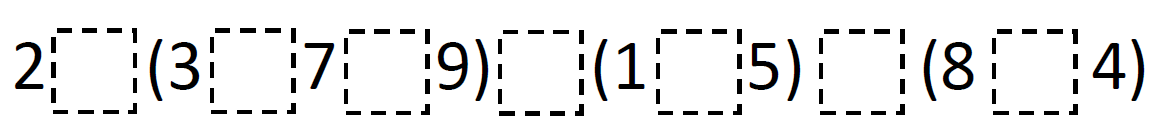Home > Grade 5 > Balanced Equations 2

# Balanced Equations 2

Directions: Use the operation symbols (+, -, x, ÷) and equal sign (=) to make a true equation. Operation symbols may be used more than once.
What is the least value for each part of the equation? What is the greatest value for each part of the equation?
Can you complete the equation with at least one of each symbol?### Hint

Where might you place the equals sign(s)?